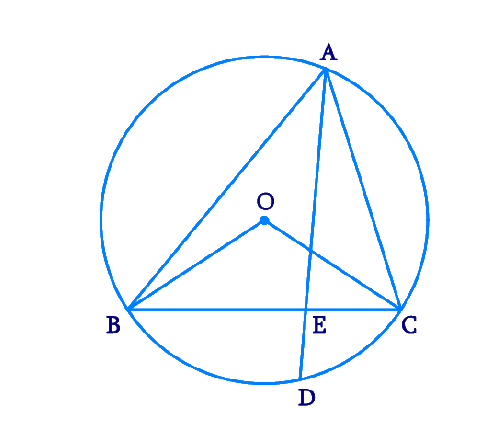# Ex.10.6 Q10 Circles Solution - NCERT Maths Class 9

Go back to  'Ex.10.6'

## Question

In any triangle \begin {align} {ABC,} \end {align} if the angle bisector of $$\angle {A}$$ and perpendicular bisector of $${BC}$$ intersect, prove that they intersect on the circumcircle of the triangle $${ABC.}$$

Video Solution
Circles
Ex 10.6 | Question 10

## Text Solution

What is known?

Bisectors of angle $$\angle {A}$$ and perpendicular bisector of $${BC}$$  intersect.

What is unknown?

Proof of the intersecting points is circumcircle.

Reasoning:

• Angles in the same segment are equal.
• The angle subtended by an arc at the centre is double the angle subtended by it at any point on the remaining part of the circle.

Steps:Let perpendicular bisector of side $${BC}$$ and angle bisector of $$\angle {A}$$ meet at point $${D.}$$ Let the perpendicular bisector of side $${BC}$$ intersect it at $${E.}$$

Perpendicular bisector of side $${BC}$$ will pass through circumcentre $${O}$$ of the circle.

$$\angle {BOC}$$ and $$\angle {BAC}$$ are the angles subtended by arc $${BC}$$ at the centre and a point $${A}$$ on the remaining part of the circle respectively. We also know that the angle subtended by an arc at the centre is double the angle subtended by it at any point on the remaining part of the circle.

\begin {align} \angle {BOC}=2 \angle {BAC}=2 \angle {A} \quad \ldots(1) \end {align}

Consider $$\angle {BOE}$$ and $$\angle {COE,}$$

\begin{align}{OE}&={OE} \quad (\text { Common }) \\ {OB}&={OC} \quad \begin{pmatrix}\! \text { Radii of} \\ \text{same circle }\!\!\end{pmatrix} \\ \angle {OEB}&=\angle {OEC} \\( \text {Each } 90^{\circ} \text { as } &{OD} \text{ perpendicular }{BC} )\end{align}

$$\therefore \Delta {BOE} \cong \angle {COE}$$ (RHS congruence rule )

$$\angle {BOE} =\angle {COE}$$ (By  CPCT) ....($$2$$)

However, \begin {align} \angle {BOE}+\angle {COE}=\angle {BOC} \end {align}

\begin{align} \;\therefore \angle {BOE}+\angle {BOE}&=2 \angle {A} \\ [\text {Using } \text{Equations } &(1) \text { and }(2)] \\ 2 \angle {BOE}&=2 \angle {A} \\ \angle {BOE}&=\angle {A}\\\angle{BOE}&=\angle {COE}\\&=\angle {A} \end{align}

The perpendicular bisector of side \begin{align}\text{BC} \end{align} and angle bisector of \begin{align}\angle {A} \end{align} meet at point \begin{align}{D.} \end{align}

\begin {align} \therefore \angle {BOD}=\angle {BOE}=\angle {A} \ldots(3) \end {align}

Since \begin{align}\angle {AD} \end{align} is the bisector of angle \begin {align} \angle {A,} \end {align}

\begin{align}\angle {BAD}&=\text { Half of angle } {A}. \\ \therefore 2 \angle {BAD}&=\angle {A} \qquad \ldots \text { (4) }\end{align}

From Equations ($$3$$) and ($$4$$), we obtain

\begin{align} \angle {BOD}=2 \angle {BAD} \end {align}

This can be possible only when point \begin{align}{BD}\end {align} will be a chord of the circle. For this, the point \begin{align}{D}\end {align} lies on the circumcircle.

Therefore, the perpendicular bisector of side \begin{align}{BC}\end {align} and the angle bisector of \begin{align} \angle {A}\end {align} meet on the circumcircle of triangle \begin{align}{ABC.}\end {align}

Video Solution
Circles
Ex 10.6 | Question 10
Learn from the best math teachers and top your exams

• Live one on one classroom and doubt clearing
• Practice worksheets in and after class for conceptual clarity
• Personalized curriculum to keep up with school